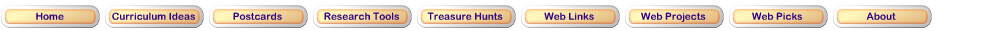Review A: MeanReview B: Rate Forget how to calculate an arithmetic mean? That's ok; just click here to remind yourself! What's a rate again? Click here to find out!Step 1: Analyzing changeReview the video from Khan Academy about Plotting a point (ordered pair). They are plotting an ordered pair on the x (horizontal) axis and y (vertical) axis of the coordinate plane.Step 2: Exploration .In this exploration, you will help Shelly and Aramis figure out how hard it is raining during different periods of the dayWeb Math The most basic plotting skill is to be able to plot x,y points. This page will help you to do that. In the box to the right, type in some x,y points like this: (1,2) or (1,2) (-4,3) (10,-6)Math is Fun Practice determining the mean.Updated March 14, 2017
© 1996 - 2009 Linda C. Joseph